Vibroengineering PROCEDIA

Published: 02 April 2020

# Large-eddy simulations of the inlet grid-generated turbulence

Zhang Zhiwei1
Wu Bo2
Zhang Liangliang3
Wang Yuanqing4
Huang Longxian5
1, 2School of Civil Engineering, Chongqing University, Chongqing, 400045, China
3City College of Science and Technology, Chongqing University, Chongqing, 400045, China
4The 5th Engineering Co., Ltd of China Railway 11th Bureau Group, Chongqing, 400037, China
5Chongqing Expressway Co., Ltd, Chongqing, 401121, China
Corresponding Author:
Wu Bo
Views 107

#### Abstract

In this paper, a new technique of turbulence generation in large eddy simulation (LES) is studied and verified. In order to generate turbulence similar to the wind tunnel test, the proposed grid inlet technique places the grid on the Inlet boundary to achieve the following effects: changing the grid size controls the turbulence integral length scale and changing the distance from inlet controls the turbulence intensity. The purpose of this paper is to explore the domain requirements of grid-inlet technology by studying the turbulence characteristics of three different grid inlets. In particular, this paper further studies the effects of domain sizes on the lateral correlation of fluctuating wind by arranging the transverse positions of monitoring points irregularly and in equal proportion. Meanwhile, the isotropic hypothesis of gird-generated turbulence is verified by power spectrum. The results show that the turbulence intensity is unaffected by the domain sizes, the larger calculation domain corresponds to the gentler changing trend of the lateral correlation of the fluctuating wind and the flow fields under the three different domain sizes basically satisfy the isotropic hypothesis. The above results are helpful for the further application of the grid inlet technique.

## 1. Introduction

Grids are typically used to experimentally generate flows with different turbulence characteristics. At present, large eddy simulation is the best method to generate turbulence. In Large Eddy Simulation, the description of turbulence characteristics is a well-known problem due to the instabilities of turbulence vortices and their temporal and spatial correlations . Pre-computation methods and synthetic inlet methods are the two typical methods to generate turbulence in In Large Eddy Simulation.

In the pre-computation method, a streamwise cyclic boundary is used to make the flow circulate on the boundary. Time data is kept in a library for application to the inlet in further simulations. Because of the time and storage required, this method is not efficient. In order to avoid the possible introduction of fictitious periodicity, shorter phase jitter pre-computation is recommended . However, to let the flow evolve into ‘real’ turbulence, a considerable simulation inlet length was found to be required. DeVilliers  describes a more efficient time and data storage method in which internal mapping planes are used to recover flow upstream of the main computational domain. In this method, there is no need to store pre-calculated data. In most pre-computation methods, it is almost impossible to generate turbulence with the specific length scale and turbulence intensity, due to the limitations of boundary layer of the domain geometry.

Carati  and Wang  studied free decaying homogeneous isotropic turbulence by synthesizing turbulence. Through modifying a random field to adapt a specified spectrum, the initial condition is generated at a single point in time. This is relatively uncomplicated, but when synthesizing inlet turbulence which is also correlated in time, difficulty begins to arise. The simplest form of a synthetic inlet is to superpose a random fluctuation on the average flow. And the fluctuations do not have structure and dissipate quickly because they are not correlated . Smirnov et al.  operated a random field to meet continuity requirements and generate a more precise description of turbulence. However, this method still does not offer satisfactory control when generating “real” turbulence. Davidson  and Fathali et al.  put forward a more advanced synthetic inlet method in which correlation filters are applied to random fields to generate turbulence with specific turbulence characteristics. However, it has been demonstrated that a reasonable inlet length is still necessary to generate turbulence with specific turbulence characteristics . It is therefore difficult to generate high-intensity turbulence through synthetic inlet methods due to turbulence dissipation.

After analyzing and comparing the above two turbulence generating methods, Tabor and Baba-Ahmadi  put forward that the most efficient turbulence generating method in LES was the internal mapping method. Based on this conclusion, a new technique of turbulence generation is proposed in which the solid patches are placed on the Inlet boundary.

By using the proposed new method, this paper develops turbulence using the projected pattern of a grid on the inlet boundary to generate grid turbulence as used in wind tunnel tests. The grid-generated turbulence has the following characteristics: changing the grid size controls the turbulence integral length scale and changing the distance from inlet controls the turbulence intensity . The purpose of this paper is to explore the domain requirements of grid-inlet technology by studying the turbulence characteristics of three different grid inlets.

## 2. Numerical model and basic methods

### 2.1. Governing equations and numerical algorithm

In this paper, numerical simulations are carried out by solving the three-dimensional unsteady incompressible governing equations of the ﬂuids. The ﬁltered continuity and Navier-Stokes equations for 3D LES with the SGS model are shown in Eq. (1). In the equations, the grid-scale turbulence is solved while the sub-grid-scale turbulence is modeled:

1

where ${u}_{i}$ and ${x}_{i}$ (1, 2, 3) are the three velocity and displacement components; $t$, $\rho$, $p$, and $\mu$ are time, density, pressure, and kinematic viscosity. The over-bar represents filtered field variable. ${\tau }_{ij}$, ${\tau }_{ij}=\stackrel{-}{{u}_{i}{u}_{j}}-{\stackrel{-}{u}}_{i}{\stackrel{-}{u}}_{j}$, is the sub-grid scale stress (SGS) , which is the product of the spatial filtration of the fluid equation and the energy exchange link between the solvable scale and the sub-grid scale, as expressed in Eq. (5):

2
${\tau }_{ij}-\frac{1}{3}{\delta }_{ij}{\tau }_{kk}=-2{\nu }_{SGS}{\stackrel{-}{S}}_{ij}={\nu }_{SGS}\left(\frac{\partial {\stackrel{-}{u}}_{i}}{\partial {x}_{j}}+\frac{\partial {\stackrel{-}{u}}_{j}}{\partial {x}_{i}}\right),$

where ${\stackrel{-}{S}}_{ij}$ is the strain rate tensor and ${\nu }_{SGS}$ is the SGS eddy viscosity ${\nu }_{SGS}={\left({C}_{S}\stackrel{-}{\Delta }\right)}^{2}\left|\stackrel{-}{S}\right|$, where ${C}_{S}$ is the Smagorinsky coefficient.

In the present study, simulations were run by using the FLUENT CFD software to solve the discrete model. A time step of 0.001 was set. The number of time steps was set to 15000; in other words, the total length is 15 s. In the process of solving the LES control equation, the Second Order discrete scheme was used for the pressure term and the Bounded Central Differentiation scheme was used for the momentum term. The numerical method is the Simple algorithm, the transient term is solved by the Bounded Second Order Implicit scheme, the sub-grid scale model is the Smagorinsky-Lilly model and the CS value is 0.1. The residual convergence standard of each physical quantity is set to 1×10-7.

### 2.2. Computational domain, mesh, and boundary conditions

Figure1 shows three different domain sizes and the positions of probes. Table1 shows the boundary conditions. In this simulation, the author uses the ANSYS ICEM to generate hexahedral structured meshes and use the ANSYS FLUNET to solve the model.

An inlet grid spacing of 40 mm, $d=$ 10 mm and a porosity of 0.56 were chosen for the sake of comparison with the simulation work done by Tom Blackmore et al. Table 2 shows the details of three different cases.

Along the flow direction of the grid inlet, 10 monitoring planes were set parallel to the grid inlet at the distances $x=$ 0.5$M$, 0.875$M$, 1.375$M$, 2.25$M$, 3.25$M$, 4$M$, 5.625$M$, 7.875$M$, 10$M$ and 13.75$M$. There were 15 monitoring points arranged unevenly in each monitoring plane; the locations of the detection points in the plane are shown in Fig. 1. In addition, a total of 10 monitoring points were set on the center line of the domain from 2 $ 20 downstream of the grid inlet.

Fig. 1Numerical domains and the positions of monitoring points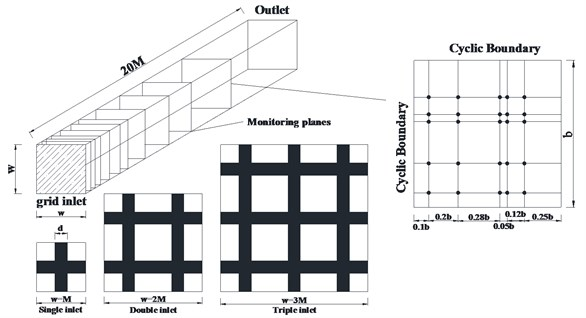Table 1Boundary conditions

 Location Boundary conditions Inlet flow Velocity-inlet,1.92 m/s Outlet Outflow Side wall Symmetry Top and bottom wall Symmetry Grid surface Non slip wall

Table 2The details of cases

 Case Mesh density Grid spacing $M$ (mm) Bar width $b$ (mm) Inlet period 1 32×32×800 40 10 Single 2 64×64×800 40 10 Double 3 96×96×800 40 10 Triple

### 2.3. Numerical validation

The turbulence intensity obtained by the present LES and the results of Tom Blackmore et al.  are presented in Fig. 2. The 10 monitoring points were set on the center line of the domain from 2 20 downstream of the grid inlet, which is the same as the case of Tom Blackmore et al. It is shown that the turbulence intensity found by the current 3D LES aligns well with that obtained by Tom Blackmore et al. (2013).

The comparison results verify the accuracy of the current 3D LES works. The turbulence characteristics of three different grid inlets are studied with respect to the turbulence intensity, turbulence integral scale and lateral correlation of the fluctuating wind so as to investigate the domain requirements for implementing the grid-inlet technique.

Fig. 2Comparisons of turbulence intensity results obtained by current LES and results of Tom Blackmore et al.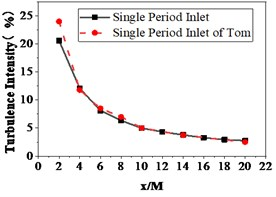a) Single period inlet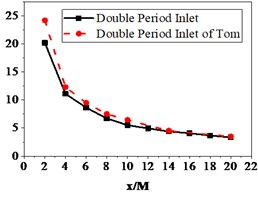b) Double period inlet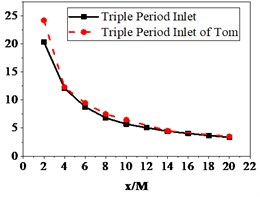c) Triple period inlet

## 3. Results and discussions

### 3.1. Turbulence intensity and turbulence integral scale

As shown in Fig. 3, the results are compared with three different grid inlet periods to study the influence of domain sizes on turbulence intensity and the turbulence integral scale along the center line from 2 20 (2 $x/M$ apart).

As shown in Fig. 3(a), the decay rate of turbulence intensity are unaffected by the domain sizes for three different grid inlet periods. However, as can be seen in Fig. 3(b), the integral scales of the three grids’ inlet flows are significantly different. The turbulence integral scale of the Single Inlet stabilizes at 0.2$M$. It is significantly smaller than the other two grids’ inlet flows because the size of the boundary is too small ($w=M$), which limits the development of the turbulent vortices. The Double Inlet’s flow integral scale stabilizes at 0.4$M$. However, the Triple Inlet’s flow integral scale continues to increase and eventually stabilizes at 0.46$M$.

After the turbulence has been fully developed, the integral scales can be listed from largest to smallest as follows: Triple Inlet, Double Inlet, Single Inlet.

Fig. 3Influence of domain sizes on the turbulence intensity and integral length scale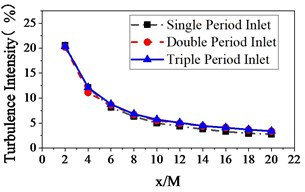a) Turbulence intensity (%)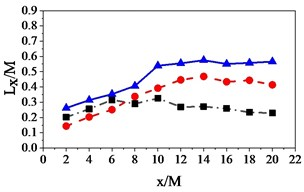b) Integral length scale (${L}_{x}/M$)

### 3.2. Fluctuating wind power spectrum

Normally, the turbulent flow generated by the grid basically satisfies the isotropic hypothesis. From the perspective of the wind spectrum, Roberts and Surry  found that the turbulent field generated by the grid could be accurately simulated by the von Kármán spectrum:

3
${S}_{u}\left({k}_{1}\right)=\frac{4{\sigma }_{u}^{2}{L}_{u}}{{\left[1+4{\pi }^{2}{\left(1.339{L}_{u}\right)}^{2}{k}_{1}^{2}\right]}^{5/6}},$

where, ${k}_{1}=n/U$ ($n$ is frequency (Hz); $U$ is mean velocity); ${L}_{u}$ is longitudinal turbulence integral scale; ${\sigma }_{u}$ is standard deviation of longitudinal fluctuating velocity.

The results are compared with three different grid inlet periods to study the influence of domain sizes on the fluctuating wind power spectrum. Fig. 4 shows the power spectrum of the longitudinal fluctuating wind for three different grid inlet periods at 2.25$x/M$, 4$x/M$ and 10$x/M$ away from the grid inlet on the center line of the computing domain. The results show that the simulations of the longitudinal fluctuating wind spectra for the three different domain sizes align well with the von Kármán spectrum. It is proven that the grid-generated turbulence basically satisfies the isotropic hypothesis and is not affected by the domain sizes.

Fig. 4Effect of inlet grid period on the isotropic hypothesis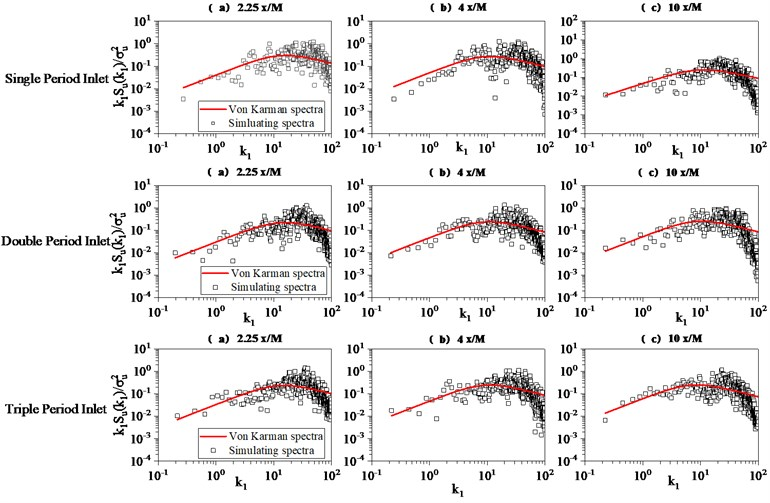### 3.3. Lateral correlation of fluctuating wind

Normally, the correlation coefficient expressed by the time domain set average can be used to describe the correlation degree of two points of fluctuating wind speed in space (Eq. (4), Eq. (5)):

4
$u\left(\stackrel{⃑}{x},t\right)=\left[{u}_{1},{u}_{2},{u}_{3}\right],$

where ${u}_{1}$, ${u}_{2}$, ${u}_{3}$ are the fluctuating wind speed in the $x$, $y$ and $z$ directions, and are the function of place and time $\left(x,y,z;t\right)$. For any two points in space, the cross-correlation function of the fluctuating components can be expressed as the second-order tensor matrix of 3×3:

5
${R}_{ij}\left(\stackrel{⃑}{r},\tau \right)=〈{u}_{i}\left(\stackrel{⃑}{x},t\right){u}_{j}\left(\stackrel{⃑}{x}+\stackrel{⃑}{r},t+\tau \right)〉,$

where, < > represents the ensemble average of two fluctuating components; $\stackrel{⃑}{r}=\left({r}_{1},{r}_{2},{r}_{3}\right)$ is the distance vector of two points in space (${r}_{1}$, ${r}_{2}$, ${r}_{3}$ is the distance along the $x$, $y$ and $z$ axes).

In this paper, the lateral correlation of fluctuating wind is studied by arranging the transverse positions of monitoring points irregularly and in equal proportion, as shown in Fig. 2. Because the domain sizes of the three kinds of grid inlets are different, dimensionless processing is used for the transverse distance through $∆y/b$, where $b$ is the width of each computational domain. As can be seen from Fig. 5(a), the Double and Triple grids show basically the same changes in the lateral correlation of the longitudinal wind. The Triple Inlet changes more gently. However, the Single grid has a larger lateral correlation. As can be seen from Fig. 5(b), the three grids exhibit basically the same changes in the lateral correlation of vertical wind when $∆y/b>\text{10}$. When $∆y/b<\text{10}$, the Single grid has a significant lateral correlation of vertical wind because the computational domain of the Single grid is so small that the turbulence can not be totally developed.

Fig. 5Influence of domain sizes on the lateral correlation of fluctuating wind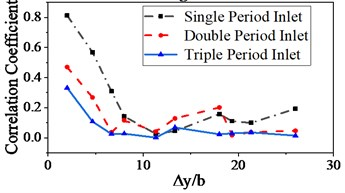a) Longitudinal wind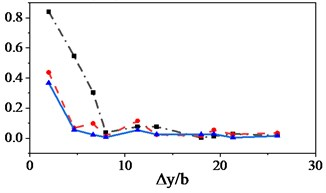b) Vertical wind

## 4. Conclusions

In this paper, the author uses the new grid inlet technique to generate isotropic turbulence with higher intensity (up to 20 %). The main conclusions of this study are as followed:

1) The turbulence intensity decay rate is unaffected by the domain sizes.

2) The turbulence integral scale is proportional to the domain sizes, with a higher a steady value for larger domain size.

3) The gird-generated turbulence basically satisfies the isotropic hypothesis, not affected by domain size.

4) When lateral distance $∆y/b<\text{10}$, the lateral correlation of fluctuating wind is inversely proportional to domain sizes; when lateral distance, the lateral correlation of three kinds of grids have basically the same change.

5) Through comparison and analysis, the triple inlet grid period (3$M$×3$M$) is the minimun domain size to make turbulence fully developed.

This study investigated the requirements of domain sizes for implementing the grid-inlet technique by studying the turbulence characteristics of three different grid inlets. By using the new grid inlet technique, the turbulence with different turbulence characteristics can be generated through deliberate selection of grid size and inlet length. The results of this paper could be helpful in the further application of the grid inlet technique.

#### Acknowledgements

This study was funded by the Natural Science Foundation of Chongqing, China, Grant No. cstc2018jscx-msyb1299, the Science and Technology Research Program of Chongqing Municipal Education Commission under the grant number KJZD-K201802501 and Graduate Scientific Research and Innovation Foundation of Chongqing under the grant number CYB17042.